# How to Calculate and Solve for the Area, Length and Height of a Trapezium | The Calculator Encyclopedia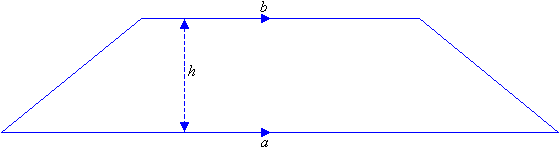The image above is a Trapezium

To compute the area of a trapezium, three essential parameter is needed and they are the length of the top side (a), length of the bottom side (b) and the height of the trapezium (h).

The formula for calculating the area of a trapezium is;

A = 0.5[a + b]h

Where;
A = Area of a trapezium
a = length of the top side of the trapezium
b = length of the bottom side of the trapezium
h = height of the trapezium

Let’s solve an example;
Find the area of a trapezium where length of top side (a) is 7 cm, length of bottom side (b) is 12 cm and height of trapezium (h) is 10 cm.

This implies that;
a = Length of top side of the trapezium = 7 cm
b = Length of bottom side of the trapezium = 12 cm
h = Height of the trapezium = 10 cm

A = 0.5[a + b]h
A = 0.5[7 + 12]10
A = 0.510
A = 95

Therefore, the area of a trapezium is 95 cm2

How to Calculate the Height of a Trapezium when the Area, Length of top side and Length of bottom side of the Trapezium is given.

The formula is h = A / 0.5(a + b)

Where;
A = Area of the trapezium
a = Length of the top side of the trapezium
b = Length of the bottom side of the trapezium

Let’s solve for an example;
Given that the length of top side (a) is 10 cm, length of bottom side (b) is 14 cm and the area of the trapezium is 20 cm2 Find the height of the trapezium?

This implies that;

A = Area of the trapezium = 20 cm2
a = Length of top side of the trapezium = 10 cm
b = Length of bottom side of the trapezium = 14 cm

h = A / 0.5(a + b)
h = 20 / 0.5(10 + 14)
h = 20 / 0.5(24)
h = 20 / 12
h = 1.667

Therefore, the height of the trapezium is 1.667 cm.

How to Calculate the Length of top side of a Trapezium when the Area of the trapezium, Length of bottom side of the Trapezium and the height of the trapezium is given.

a = 2A / h – b

where;

A = Area of the trapezium
b = Length of the bottom side of the trapezium
h = Height of the trapezium

Let’s solve an example;
Find the length of top side of a trapezium when the height of the trapezium is 14 cm, length of bottom side of the trapezium is 16 cm and area of the trapezium is 240 cm2

This implies that;

h = Height of the trapezium = 14 cm
b = Length of bottom side of the trapezium = 16 cm
A = Area of the trapezium = 240 cm2

a = 2A / h – b
a = 2(240) / 14 – 16
a = 480 / 14 – 16
a = 34.26 – 16
a = 18.256

Therefore, the length of top side of the trapezium is 18.256 cm.

How to Calculate the Length of bottom side of a Trapezium when the Area of the trapezium, Length of top side of the Trapezium and the height of the trapezium is given.

b = 2A / h – a

where;

A = Area of the trapezium
a = Length of the top side of the trapezium
h = Height of the trapezium

Let’s solve an example;
Find the length of the bottom side of a trapezium when length of the top side of the trapezium is 20 cm, height of the trapezium is 25 cm and area of the trapezium is 300 cm2

This implies that;
a = Length of top side of the trapezium = 20 cm
h = height of the trapezium = 25 cm
A = Area of the trapezium = 300 cm2

b = 2A / h – a
b = 2(300) / 25 – 20
b = 600 / 25 – 20
b = 24 – 20
b = 4

Therefore, the length of bottom side of the trapezium is 4 cm.

Nickzom Calculator – The Calculator Encyclopedia is capable of calculating the area of a trapezium.

To get the answer and workings of the area of a trapezium using the Nickzom Calculator – The Calculator Encyclopedia. First, you need to obtain the app.

You can get this app via any of these means:

To get access to the professional version via web, you need to register and subscribe for NGN 1,500 per annum to have utter access to all functionalities.
You can also try the demo version via https://www.nickzom.org/calculator

Once, you have obtained the calculator encyclopedia app, proceed to the Calculator Map, then click on Mensuration under the Mathematics section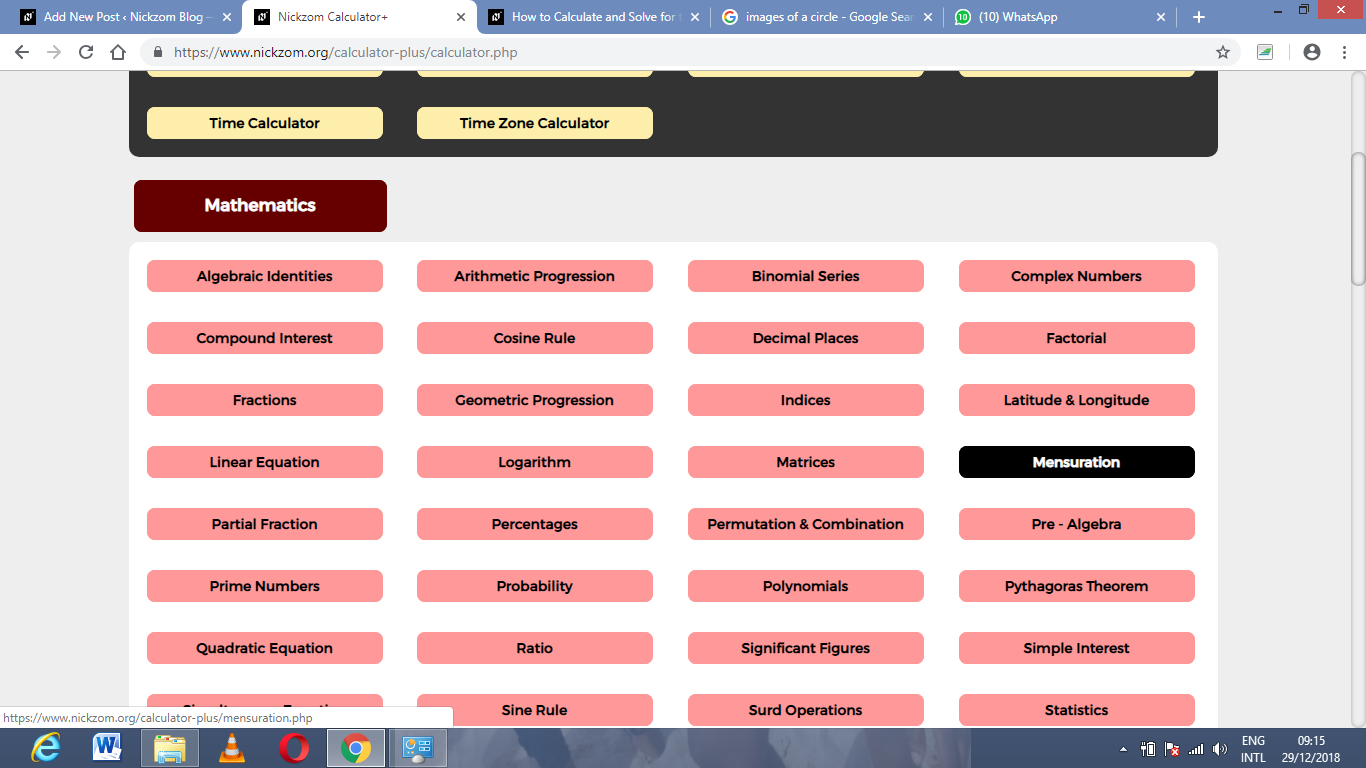Now, click on Area of a trapezium under Mensuration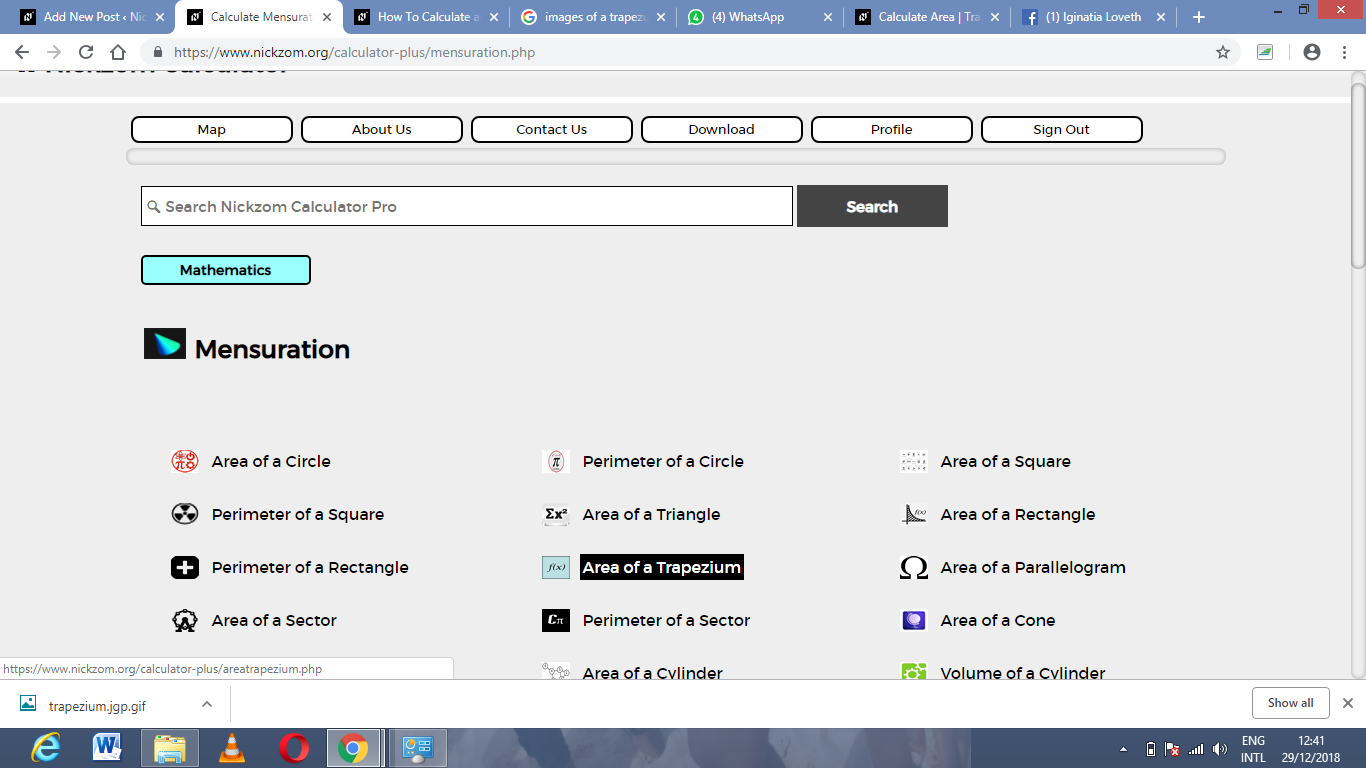The screenshot below displays the page or activity to enter your value, to get the answer for the area of a trapezium according to the respective parameters which is the length of top side of the trapezium, length of bottom side of the trapezium and height of the trapezium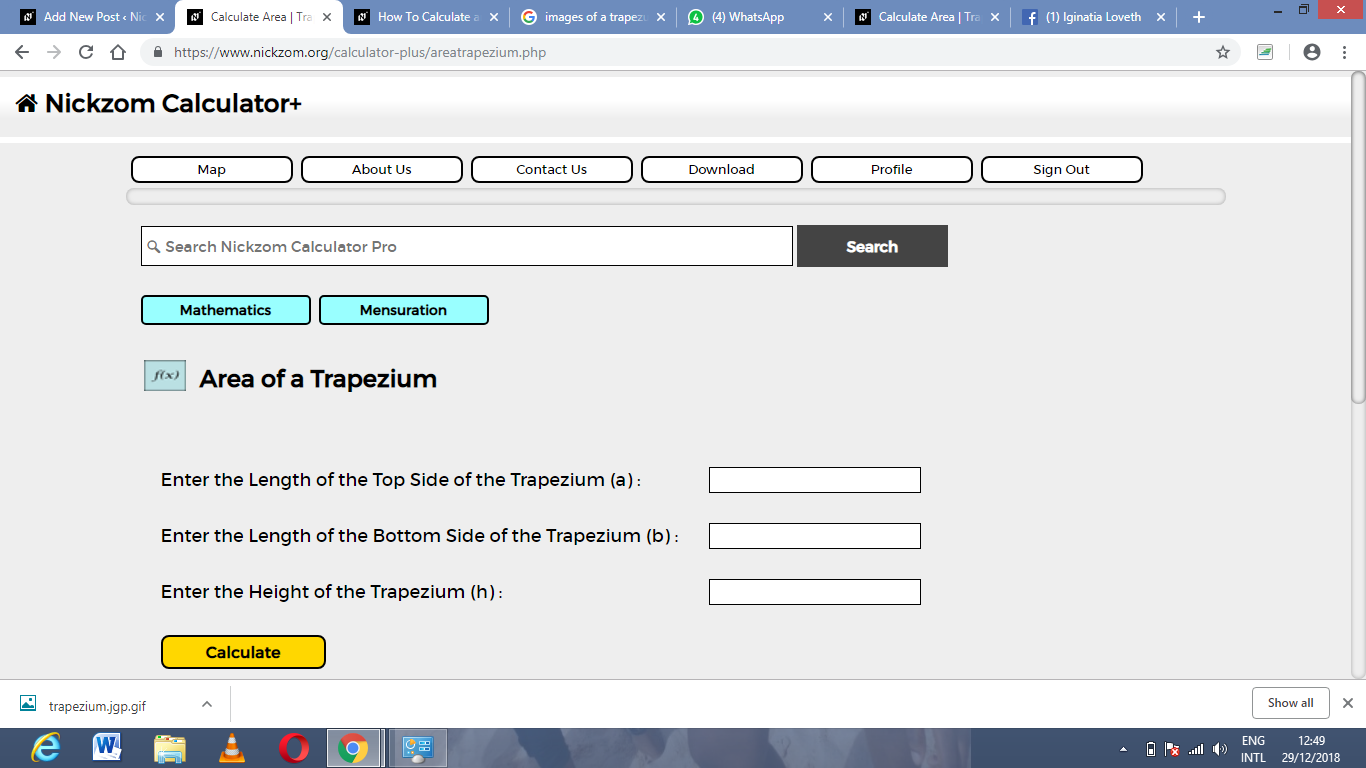Now, enter the values appropriately and accordingly for the parameters as required by the example above where the length of the top side of the trapezium is 7 cm, length of the bottom side of the trapezium is 12 cm and height of the trapezium is 10 cm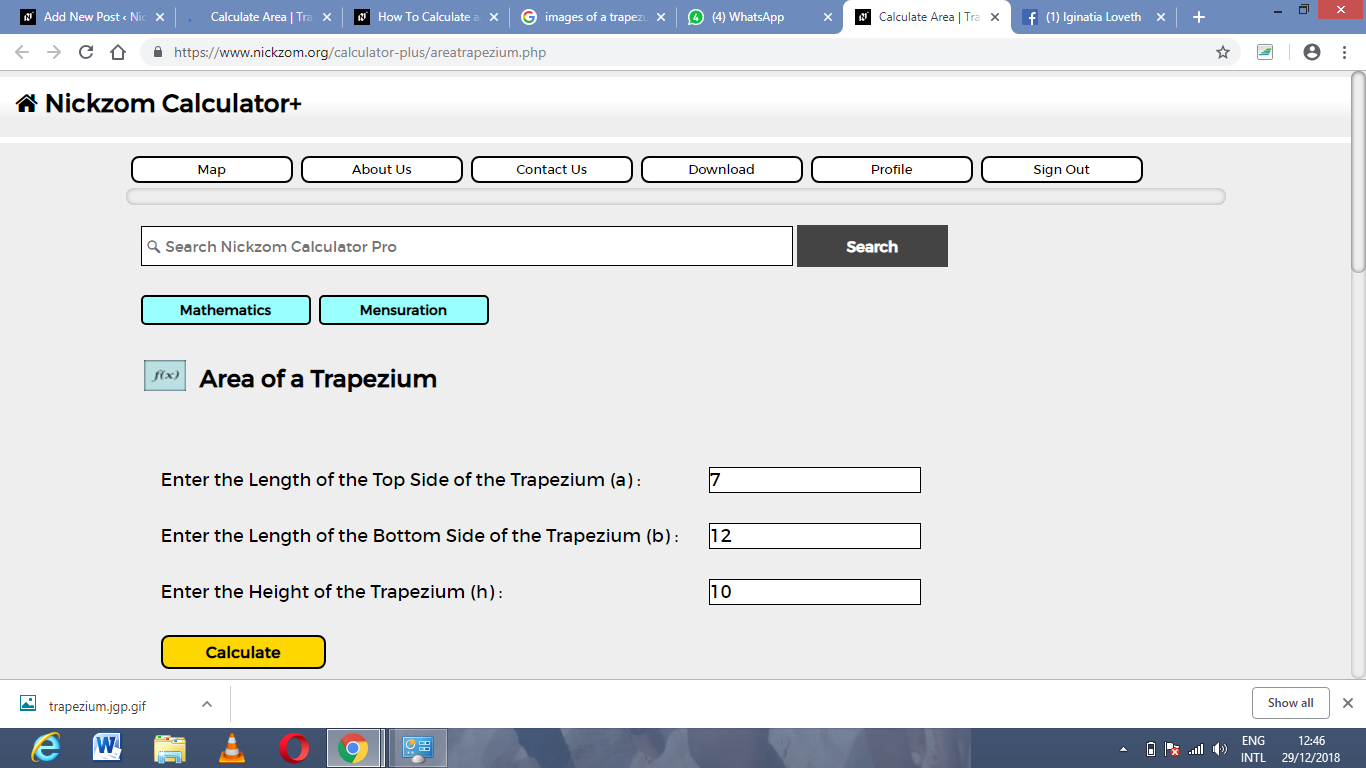Finally, click on Calculate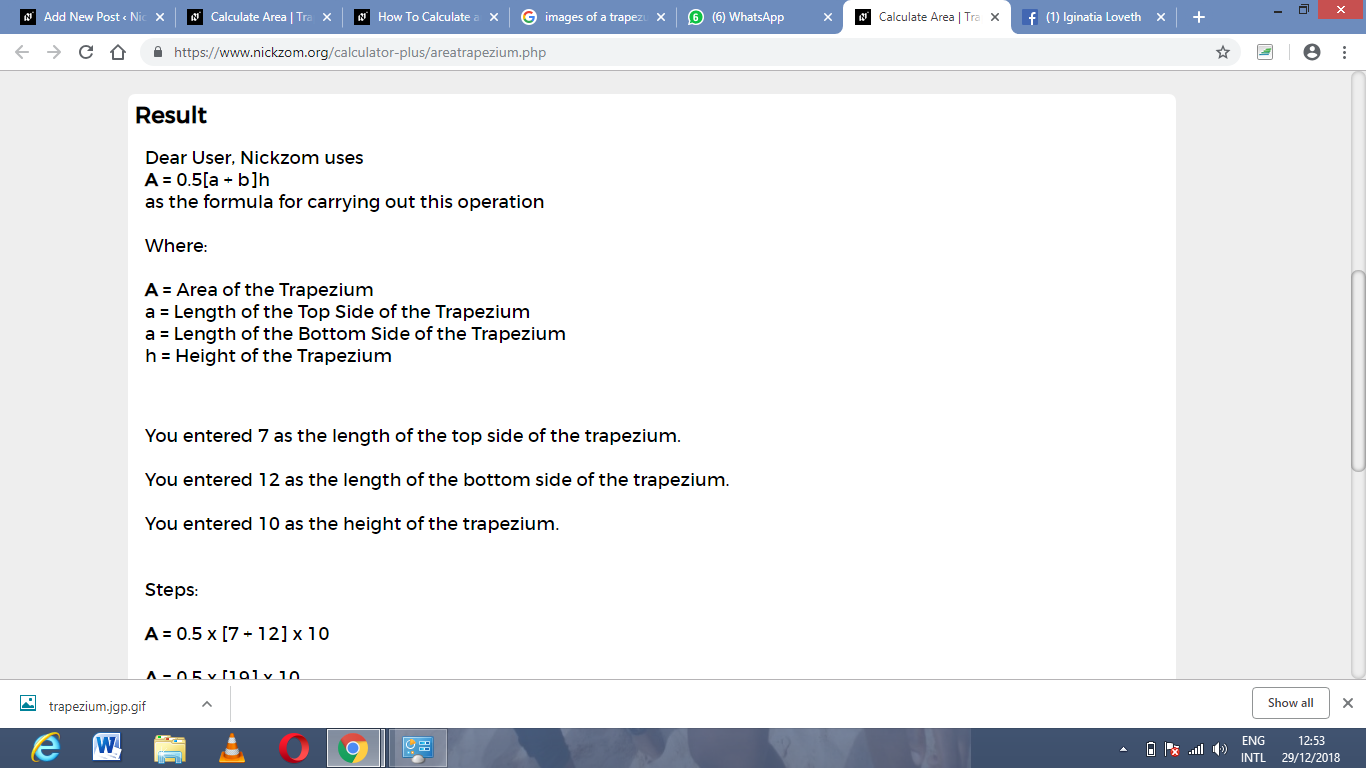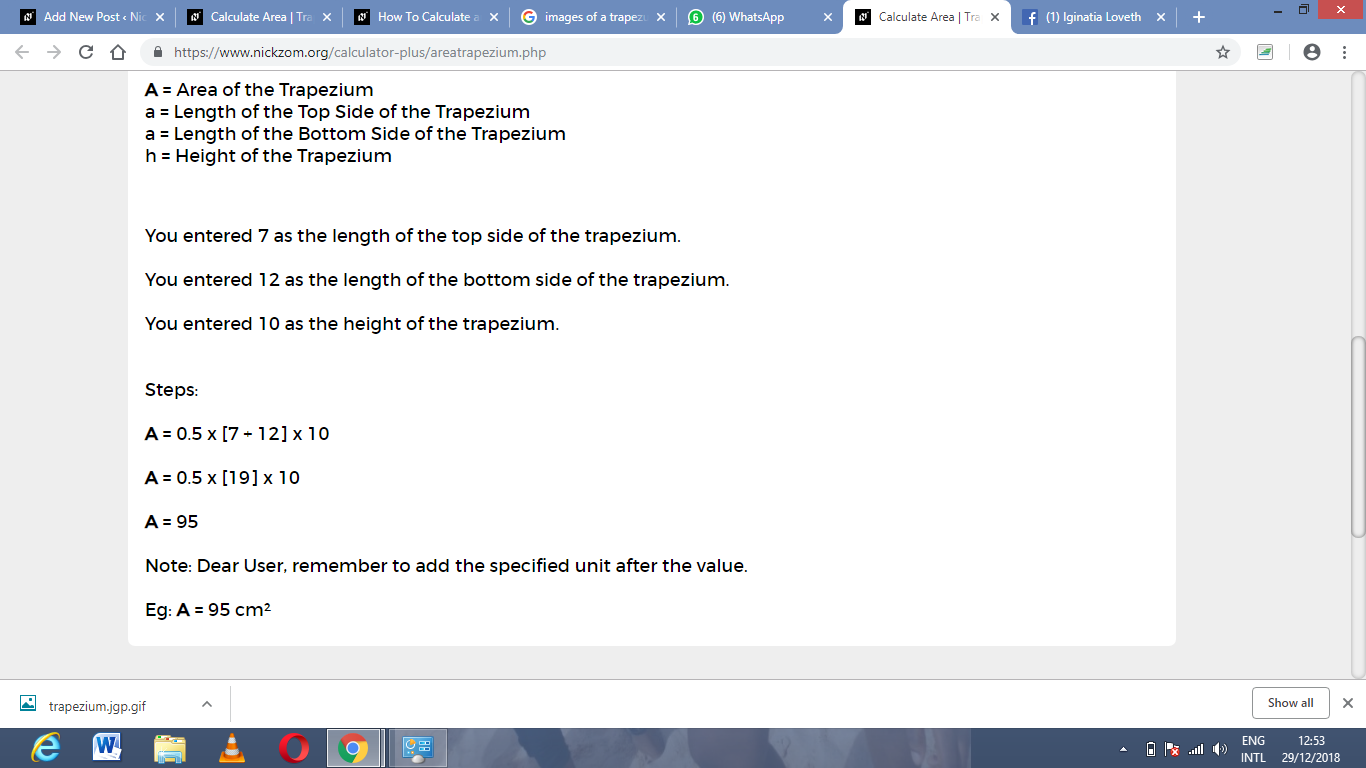As you can see from the screenshot above, Nickzom Calculator – The Calculator Encyclopedia solves for the area of a trapezium and presents the formula, workings and steps too.